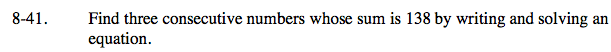### Home > CAAC > Chapter 8 > Lesson 8.1.4 > Problem8-41

8-41.Write an equation to solve the problem.
first number = x
second number = x + 1
third number = x + 2
x + (x + 1) + (x + 2) = 138